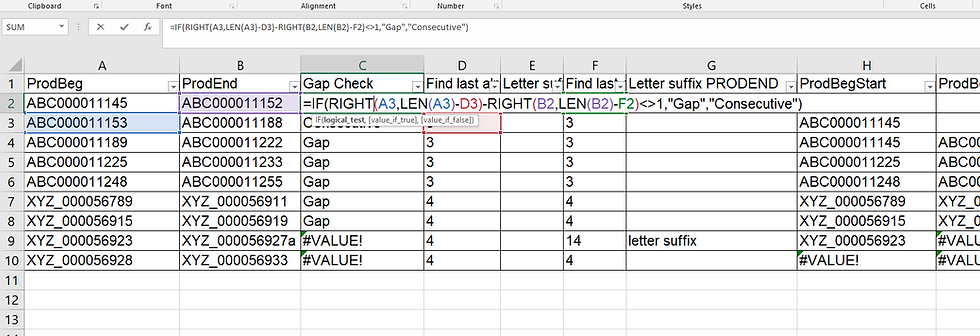top of pageSearch

# Bates number gap checker

The Excel spreadsheet posted below tonight can be used to generate a list of Bates number ranges, listing consecutively numbered ranges in a long list that has gaps, and includes ranges from different party's productions.

Enter your list of beginning and ending Bates numbers in columns A and B. Be sure they are sorted in numerical order. The formula in column C checks to see where the difference between the number (minus the Bates prefix) in column A is a different than one whole number, but it only checks the characters in each cell after the last non-numerical character appears.

=IF(RIGHT(A3,LEN(A3)-D3)-RIGHT(B2,LEN(B2)-F2)<>1,"Gap","Consecutive")The formula is designed to do this because Bates numbers always end with numbers of several digits and almost always contain prefixes with letters, hyphens, underscores, spaces, and other non-numerical characters, and can sometimes contain numbers as well such as 'ACME-2004_00099454256', or '3M 0006532'.

The formulas in columns D and F search for the position where the last non-number appears, and the formula in column C references the result of those formulas.

=LOOKUP(2,1/(ISNUMBER(SEARCH(MID(A2,ROW(INDIRECT("1:"&LEN(A2))),1),"abcdefghijklmnopqrstuvwxyz@#\$%^&+ _-:|.—"))),ROW(INDIRECT("1:"&LEN(A2))))Thanks to tigeravatar for posting this LOOKUP formula here. Note that the formula will fail if the Bates prefix ends with any character other than these: abcdefghijklmnopqrstuvwxyz@#\$%^&+ _-:|.—

It can used for more than one purpose where you need to find the last occurrence of a character or characters in a cell.

The formulas in columns E and G flag where a Bates number ends with a letter suffix. In these cases the Bates ranges will have to be manually checked.

The formula in column H checks for where the Bates prefix in column A differs from the preceding Bates prefix, and enters a new Bates number where the formula in column C indicates a gap appears.

=IF(LEFT(A7,D7)<>LEFT(A6,D7),A7,IF(C6="Gap",A7,H6))The formula in the last column enters the ending Bates number for each consecutive range. Simply filter in this column to get the complete ranges for all Bates numbers in H and I, but be sure to manually correct the very last Bates number.Bates Gap Checker
.xlsx